# KSEEB Solutions for Class 9 Maths Chapter 2 Introduction to Euclid Geometry Ex 2.1

KSEEB Solutions for Class 9 Maths Chapter 2 Introduction to Euclid Geometry Ex 2.1 are part of KSEEB Solutions for Class 9 Maths. Here we have given Karnataka Board Class 9 Maths Chapter 2 Introduction to Euclid Geometry Exercise 2.1.

## Karnataka Board Class 9 Maths Chapter 2 Introduction to Euclid Geometry Ex 2.1

Question 1.
Which of the following statements are true and which are false? Give reasons for your answers.
(i) Only one line can pass through a single point.
False. A number of lines can pass through a single point.

(ii) There is an infinite number of lines which pass through two distinct points.
False. Because there is a unique line that passes through two points.

(iii) A terminated line can be produced indefinitely on both sides.
True. A terminated line can be produced indefinitely on both sides.

(iv) If two circles’ are equal, then their radii are equal.
True. Because the circumference of equal circles is equal their distance is the same from centre. Hence radii of two circles are equal.

(v) In Fig 2.9, if AB = PQ and PQ = XY, then AB = XY.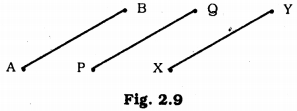True. Because E.g., If AB = 4 cm, PQ = 4 cm., then, AB = PQ = 4 cm.
PQ = XY = 4 cm.
∴ AB = PQ = XY
∴ AB = XY.

Question 2.
Give a definition for each of the following terms. Are there other terms that need to be defined first? What are they, and how might you define them?
(i) parallel lines
(ii) perpendicular lines
(iii) line segment
(iv) the radius of a circle
(v) square.
(i) Parallel Lines :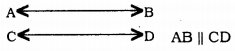If endpoints of two straight lines do not meet even if they are produced on both sides, they are called parallel lines.
Before the definition concept of point, a straight line is necessary.
Point: A point is that which has no part.
Straight-line: A straight line is a line which lies evenly with the points on itself.

(ii) Perpendicular Lines: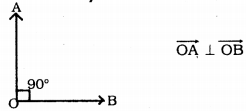If Angle between $$\overrightarrow{\mathrm{OA}}$$ and $$\overrightarrow{\mathrm{OB}}$$ is 90°, then only lines are perpendicular mutually.
Before the definition concept of Ray and Angle is necessary.
Ray: Joining one endpoint and the non-end point is called Ray.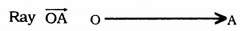Angle: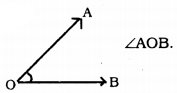Ray $$\overrightarrow{\mathrm{OA}}$$ and Ray $$\overrightarrow{\mathrm{OB}}$$ revolve and forms an angle.

(iii) Segment: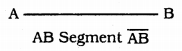The segment is formed when two endpoints are joined.
Here we must know the point and straight line.
Point: It has only dimension, no length, breadth, and thickness.
A – Starting point
B – Endpoint
AB – Segment
Straight-line: When two endpoints of a segment is produced on both sides, we get a straight line.

iv) The radius of a Circle :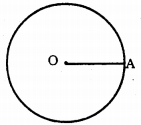A circle is a set of points that moves from a fixed point.
That equal distance is the radius of the circle.
‘O’ – Fixed point
OA – radius of the circle.
Necessary terms – Point, Segment.

v) Square :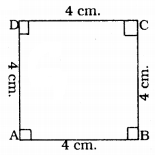A Square is a figure in which all sides and angles are equal.
AB = BC = CD = DA = 4 cms.
∠A = ∠B = ∠C = ∠D = 90°.
Quadrilateral In a plane, if 3 points in 4 points are non-collinear and meet in different points, such a figure is called a quadrilateral.
Angle: ‘O’ is common point between $$\overrightarrow{\mathrm{OA}}$$ and $$\overrightarrow{\mathrm{OB}}$$ Such figure is called an angle.

Question 3.
Consider two ‘postulates’ given below :
(i) Given any two distinct points A and B, there exists a third point C which is in between A and B.
(ii) There exist at least three points that are not on the same line.
Do these postulates contain any undefined terms? Are these postulates consistent? Do they follow from Euclid’s postulates? Explain.
There are many undefined terms for students.
They are consistent because they deal with two different situations.
(i) When two points A and B are given, C is in between two.
(ii) When two points A and B are given take point C which passes through A and B.
These postulates do not follow from Euclid’s postulates. But they follow Axiom 2.1

Question 4.
If a point C lies between two points A and B such that AC = BC, then prove that $$A C=\frac{1}{2} A B$$. Explain by drawing the figure.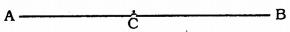Data: C lies between A and B such that AC = BC.
To Prove: AC = $$\frac{1}{2}$$AB
Proof: In this figure AC = BC.
AC + AC = BC + AC (equals are added to equals)
2AC = BC + AC
2AC = AB (∵ BC + AC = AB)
∴ AC = $$\frac{1}{2}$$AB.

Question 5.
In Question 4, point C is called a mid¬point of line segment AB. Prove that every line segment has one and only one mid-point.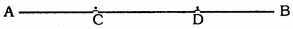Let C and D are two points lies on AB.
C is the mid-point of AB.
AC = BC
AC + AC = BC + AC
2AC = AB → (i)
D is the mid-point of AB,
From equations (i) and (ii),
Then C and D are not different, they are the same.
∴ “Every segment has one and only one mid-point.”

Question 6.
In Fig. 2.10, if AC = BD, then prove that AB = CD.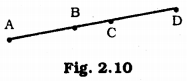Data: In this figure AC = BD.
To Prove : AB = CD
Proof: AC = BD (Data) → (i)
But, AC = AB + BC
and BD = BC + CD.
Substituting the value of (i)
AC = BD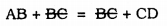∴ AB = CD.

Question 7.
Why is Axiom 5, in the list of Euclid’s axioms, considered a ‘universal truth’ ? (Note that the question is not about the fifth postulate).